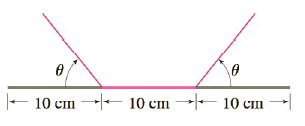Chapter 3.7, Problem 76E

Chapter
Section
Textbook Problem

# A rain gutter is to be constructed from a metal sheet of width 30 cm by bending up one-third of the sheet on each side through an angle θ . How should θ be chosen so that the gutter will carry the maximum amount of water?To determine

To find:

To choose the value of θ so that the gutter carries the maximum amount of water

Explanation

1) Concept:

First Derivative Test for Absolute Extreme Values:

Suppose that c is a critical number of a continuous function f defined on an interval.

(a) I f'x>0 for all x<c and f'x<0 for all x>c then fc is the absolute maximum value of f.

(b) If  f'x<0, 0 for all x<c and f'x>0 for all x>c then fc is the absolute minimum value of f.

2) Calculation:

From the figure,

sinθ=h10, cosθ=d10

h=10sinθ & d=10cosθ

So the amount of water is a function of θ

Aθ=10h+212dh

Substitute the values of d & h

Aθ=10·10sinθ+10cosθ·10sinθ

Aθ=100sinθ+cosθsinθ

For 0θπ2

Differentiate with respect to θ

A'(θ)=100(cosθ-sin2θ+cos2θ)

A'(θ)=100(cosθ-1+cos2θ+cos2θ)

A'θ=100cos2;

### Still sussing out bartleby?

Check out a sample textbook solution.

See a sample solution

#### The Solution to Your Study Problems

Bartleby provides explanations to thousands of textbook problems written by our experts, many with advanced degrees!

Get Started

#### In Exercises 2934, rationalize the denominator of each expression. 33. a+bab

Applied Calculus for the Managerial, Life, and Social Sciences: A Brief Approach

#### The zeroes of a function correspond to what feature of its graph?

Mathematical Applications for the Management, Life, and Social Sciences

#### 0 1 ∞

Study Guide for Stewart's Multivariable Calculus, 8th

#### Simplify each expression. i26

College Algebra (MindTap Course List)

#### Identify the threats to internal validity for pre-post designs.

Research Methods for the Behavioral Sciences (MindTap Course List)